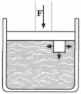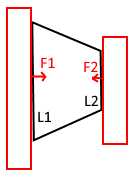# Pressure on a Piston

CGandC

## Homework Statement

Suppose there is a tank filled with water and a piston of area S exerts a force F on the water.Suppose I divide the water boundary touching the piston to - N small equal " square " molecules.
Then , the force on the upper face of each molecule is F/N .
Also, the area of the upper face of each molecule is of length S/N .
Thus , the pressure on each molecule touching the piston is : ## P = \frac{F/N}{S/N} = \frac{F}{S} ##
And this is the same pressure that each molecule exerts on the piston

Question: If ## P = \frac{F}{S} ## is also the pressure that each molecule ( touching the piston ) exerts on the piston, then , if I sum the pressures exerted by each such molecule on the piston, I get:

## P = \frac{F}{S} + \frac{F}{S} + ... + \frac{F}{S} = \frac{N*F}{S} ##

which contradicts conservation of energy and also because it just seems wrong because I was told the pressure on the piston is just : ## P = \frac{F}{S} ## and not ## P = \frac{N*F}{S} ##

Why is there contradiction of conservation of energy and why the pressure on the piston is not ## P = \frac{N*F}{S} ##?

## The Attempt at a Solution

#### Attachments

Staff Emeritus
Homework Helper
Gold Member
Pressure is an intensive quantity, not an extensive one. You sum forces, not pressures.

Homework Helper
Gold Member
2022 Award
You sum forces, not pressures.
Well, you can sum pressures if they all act on the same patch of surface, but then we would have pressure NF/S acting on area S/N for a total force of F and an average pressure over S of F/S.

CGandC
Pressure is an intensive quantity, not an extensive one. You sum forces, not pressures.

So if for example, I have a trapezoid block of mass and two pistons are acting on either side of it, each exerting force F1 and F2 upon the faces L1 and L2 respectively, as shown in the figure below:Then, I think the total pressure on the trapezoid should be:
## P = \frac{F_1}{L_1} + \frac{F_2}{L_2} ##
But according to what you said, pressure is an intensive property so summation like this cannot be done.

So my question is: what should be the total pressure acting on the trapezoid?

Staff Emeritus